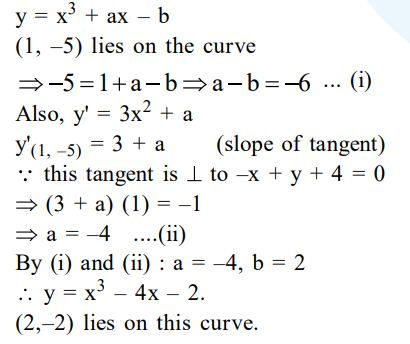# If the tangent to the curveQuestion:

If the tangent to the curve, $y=x^{3}+a x-b$ at the point $(1,-5)$ is perpendicular to the line, $-x+y+4=0$, then which one of the following points lies on the curve ?

1. $(-2,2)$

2. $(2,-2)$

3. $(2,-1)$

4. $(-2,1)$

Correct Option: , 2

Solution: